# chromatic number, fractional power of graph, clique number

## Vertex Coloring of graph fractional powers ★★★

Conjecture   Letbe a graph andbe a positive integer. Thepower of, denoted by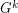, is defined on the vertex set, by connecting any two distinct verticesand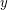with distance at most. In other words,. Alsosubdivision of, denoted by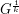, is constructed by replacing each edgeofwith a path of length. Note that for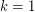, we have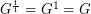.
Letbe a graph and. The graph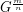is defined by thepower of thesubdivision of. In other words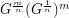.
Conjecture. Letbe a connected graph withandbe a positive integer greater than 1. Then for any positive integer, we have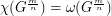.